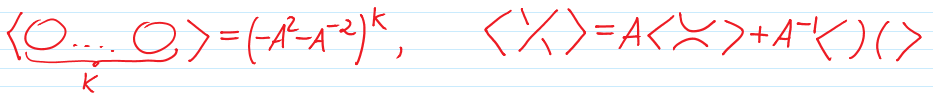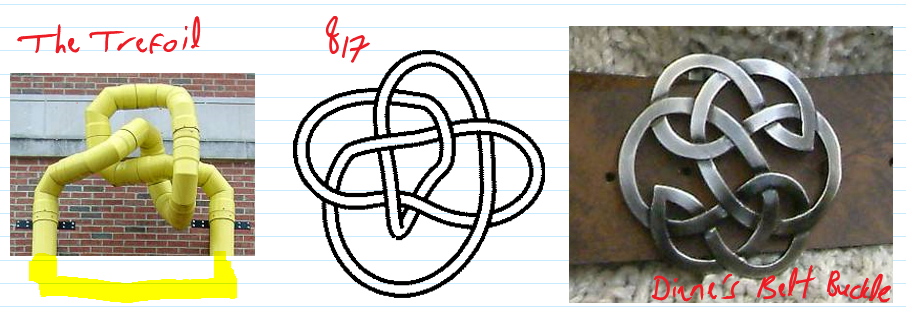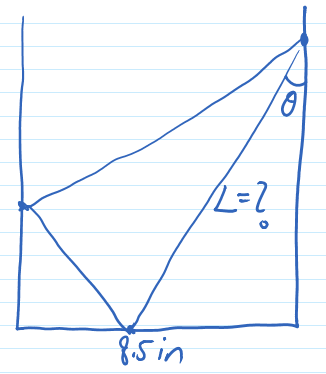$\def\bbN{{\mathbb N}} \def\bbQ{{\mathbb Q}} \def\bbR{{\mathbb R}} \def\bbZ{{\mathbb Z}}$
 © | Dror Bar-Natan: Classes: 2014-15: Math 475 - Problem Solving Seminar: (20) Next: Blackboards for Tuesday February 3 Previous: Quiz #4 - Choose an Effective Notation

# Handout for February 3, "Choose an Effective Notation"

Reading. Section 1.5 of Larson's textbook.

Dror's Favourite "Choose an Effective Notation" problem is more a computer problem than a math problem, yet it is pretty and it will make us look at some pretty (and significant!) mathematics.

The Kauffman Bracket $\langle D\rangle$ of a knot diagram $D$ is defined by the following recursive relations:(where $A$ is some variable).

Remark. The celebrated "Jones Polynomial" of $D$ is very easily defined from $\langle D\rangle$, $J(D) = \left.(-A^3)^{w(D)}\frac{\langle D\rangle}{-A^2-A^{-2}}\right|_{A\to q^{1/4}},$ where $w(D)$ is the writhe of $D$, the difference between the number of right-handed and left-handed crossings in $D$. Yet this matters not for us today.

Homework Problem (not to be submitted, yet fame for an excellent solution). Write a very short computer program that will be able to compute the Kauffman bracket (and hence the Jones polynomial) of the following three knots:Problem 1 (Larson's 1.5.7). The bottom of this handout (width 8.5in) is folded up and to the left, so that the bottom right corner would align with the left edge of this page. Given the angle of the fold $\theta$, what is the length of the crease $L$? See the figure on the right.

Problem 2 (Larson's 1.5.8). Let $P_1,P_2,\ldots,P_{12}$ be the vertices of a regular 12-gon. Are the diagonals $P_1P_9$, $P_2P_{11}$ and $P_4P_{12}$ concurrent?

Problem 3 (Larson's 1.5.2).

1. If $n\in\bbN$ and $2n+1$ is a square, show that $n+1$ is the sum of two successive squares.
2. If $n\in\bbN$ and $3n+1$ is a square, show that $n+1$ is the sum of three squares.